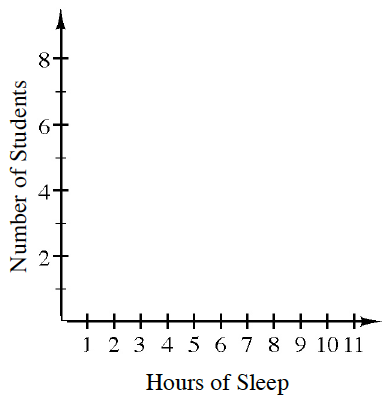### Home > CC3MN > Chapter 1 > Lesson 1.2.2 > Problem1-59

1-59.

Kelly asked some of her classmates how many hours of sleep they get on school nights. Here are the results:

$6,8,7\frac{1}{2},9,8,8,8,$ $9,9,10,6,8\frac{1}{2},9,7,8$

Copy the set of axes below and create a histogram for the data. (Refer to the glossary in the eBook for assistance, if needed.)Determine bins appropriate to your data (how wide each bar will be). For this problem use one hour bins.

Think of each bin as containing all the values between the number on the left and the number on the right. For example, a value of $6.5$ would be counted with the bar between $6$ and $7$, but a value of $7.0$ would be counted with the bar between $7$ and $8$.

Count the number of values in each bin. Draw a bar for each bin. The height of the bar corresponds to the number of values in the bin.